# Quant (DI, DS) Quiz for IBPS CLERK MAINS and Canara bank po | 20th December 2018

Quant Quiz to improve your Quantitative Aptitude & Data Interpretation. For upcoming banking exams like SBI PO, SBI Clerk, IBPS PO, IBPS Clerk etc.

Directions (1 to 5): Given below are the two pie charts. First Pie chart shows the percentage distribution of a man total income for seven year from year 2009 to 2015

Second pie chart shows the percentage distribution of his total expenditure from year 2009 to 2015

Note  Income = Expenditure + Profit

Ratio between total income and total expenditure for seven year is 91 : 73

Difference between income and expenditure in 2011 is 300.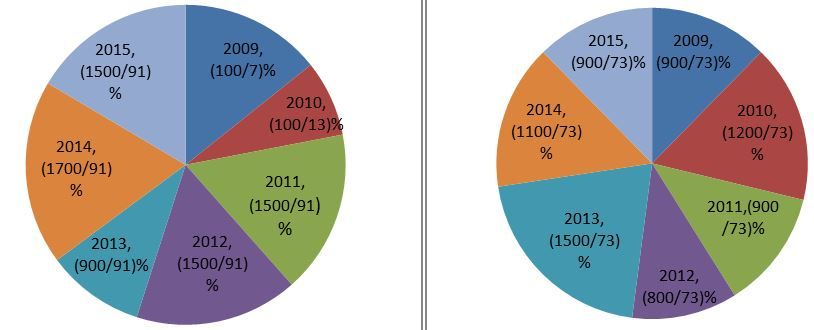Q1. Profit earned by man in year 2011 and 2012 together is what percent less or more than the profit earned by man in year 2014 and 2015 together.Q2. What is the ratio of maximum profit for any year to the maximum loss for any year, if income = expenditure – loss?

(a) 3 : 5

(b) 4 : 5

(c) 2 : 1

(d) 7 : 6

(e) 6 : 1

Q3. Average of income for year 2011, 2014 and 2015 is what percent more or less than the average of expenditure for years 2010 2013 and 2015?

(a) 28%

(b) 29%

(c) 30.5%

(d) 31.5%

(e) 28.5%

Q4. If income in year 2016 is decreased by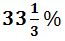as compared to previous year and ratio of expenditure for year 2015 and 2016 is 9 : 5 then profit in year 2016 changes by what percent as compared to previous year?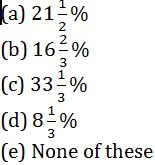Q5. For how many years, expenditure of person is more than the income of the person and sum of difference between expenditure and income for those two years is what percent of sum of difference between Income and expenditure for remaining years.

(a) 28%

(b) 32%

(c) 38%

(d) 26%

(e) 42%

Directions (6 to 10): Each of these examples is followed by three statements. You have to study the question and all the three statements given to decide whether any information provided in the statement(s) is redundant and can be dispensed with while answering the given question.

Q6. What is the area of ∆ABC, right angled at B.

I. Length of AC is 5 cm.

II. Perimeter of the triangle is four times of BC.

III. ∠BCA = 60°

(a) Only I

(b) Only II

(c) Only III

(d) Either II or III

(e) All I, II and III are required

Q7. At what time will the train reach city X from City Y?

I. The train crosses another train of equal length of 200 m, running opposite in direction in 15 s,

II. The train leaves city Y at 7.15 am for city X situated at a distance at 560 km.

III. The 300 m long train crosses a signal pole in 10 s.

(a) Only I

(b) Only II

(c) Only III

(d) Either II or III

(e) All I, II and III are required to answer the question

Q8. What will be the sum of the ages of father and the son after five years?

I. Father’s present age is twice the present age of son.

II. After ten years, the ratio of father’s age to the son’s age will become 12 : 7

III. Five years ago, the difference between the father’s age and son’s age was equal to son’s present age.

(a) Only I or II

(b) Only II or III

(c) Only I or III

(d) Only I

(e) Only I or II or III

Q9. 12 men and 8 women can complete a piece of work in 10 days. How many days will it take for 15 men and 4 women to complete the same work?

I. 15 men can complete the work in 12 days.

II. 15 women can complete the work in 16 days.

III. The amount of work done by a woman is three fourth of the work done by a man in one day.

(a) Only I

(b) Only III

(c) Only II or III

(d) Only I or II or III

(e) Any two of the three

Q10. What is the length of a running train P crossing another running train Q.

I. These two trains take 18 s to cross each other.

II. These trains are running in opposite direction.

III. The length of the train Q is 180 m.

(a) Only I

(b) Only II

(c) Only III

(d) All I, II and III are required

(e) Cannot be answered even with the help of three statements

## Solution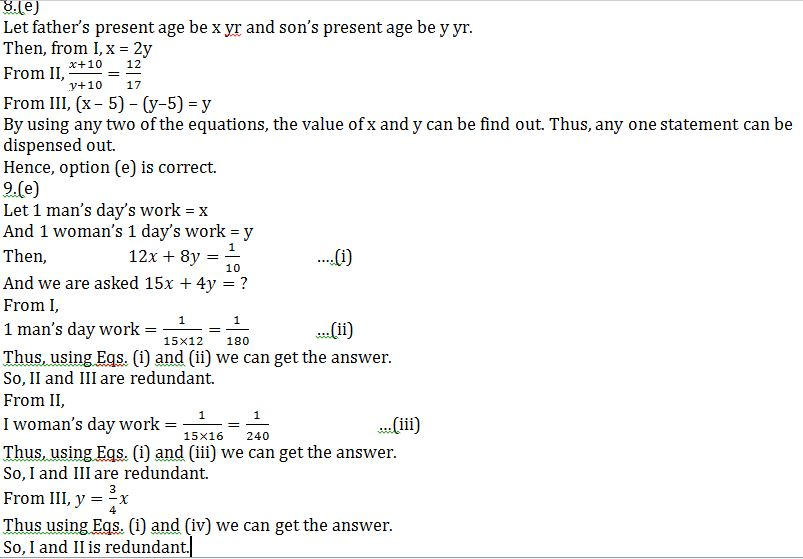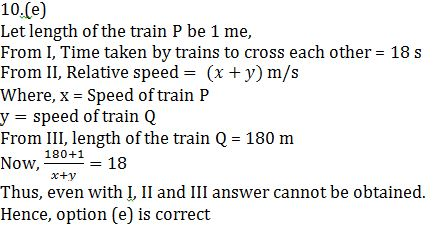## WhatsApp Group Join here

Mail us at : ambitiousbaba1@gmail.com